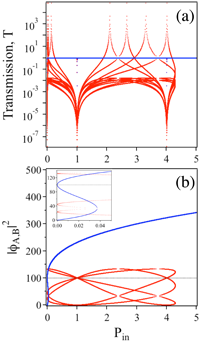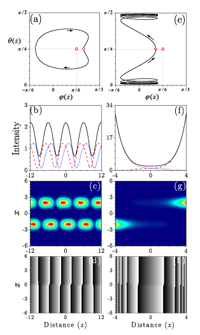## Magnetoelectric Effects in Local Light-Matter Interactions

Konstantin Y. Bliokh, Yuri S. Kivshar, Franco Nori

We study the generic interaction of a monochromatic electromagnetic field with bi-isotropic nanoparticles. Such an interaction is described by dipole-coupling terms associated with the breaking of dual, P- and T-symmetries, including the chirality and the nonreciprocal magnetoelectric effect. We calculate absorption rates, radiation forces, and radiation torques for the nanoparticles and introduce novel characteristics of the field quantifying the transfer of energy, momentum, and angular-momentum in these interactions. In particular, we put forward the concept of ‘magnetoelectric energy density’, quantifying the local PT-symmetry of the field. Akin to the ‘super-chiral’ light suggested recently for sensitive local probing of molecular chirality [Phys. Rev. Lett. 104, 163901 (2010); Science 332, 333 (2011)], here we describe a complex field for sensitive probing of the nonreciprocal magnetoelectric effect in nanoparticles or molecules.

http://arxiv.org/abs/1312.4325

Optics (physics.optics); Other Condensed Matter (cond-mat.other); Quantum Physics (quant-ph)

## Pseudo Parity-Time Symmetry in Optical Systems

Xiaobing Luo, Honghua Zhong, Jiahao Huang, Xizhou Qin, Qiongtao Xie, Yuri S. Kivshar, Chaohong Lee

We introduce a novel concept of the {\em pseudo} parity-time ($$\mathcal{PT}$$) symmetry in periodically modulated optical systems with balanced gain and loss. We demonstrate that whether the original system is $$\mathcal{PT}$$-symmetric or not, we can manipulate the property of the $$\mathcal{PT}$$ symmetry by applying a periodic modulation in such a way that the effective system derived by the high-frequency Floquet method is $$\mathcal{PT}$$ symmetric. If the original system is non-$$\mathcal{PT}$$ symmetric, the $$\mathcal{PT}$$ symmetry in the effective system will lead to quasi-stationary propagation that can be associated with the \emph{pseudo $$\mathcal{PT}$$ symmetry}. Our results provide a promising approach for manipulating the $$\mathcal{PT}$$ symmetry of realistic systems.

http://arxiv.org/abs/1302.1091
Optics (physics.optics); Quantum Physics (quant-ph)

## Discrete vortex solitons and PT symmetry

Daniel Leykam, Vladimir V. Konotop, Anton S. Desyatnikov

We study the effect of lifting the degeneracy of vortex modes with a PT symmetric defect, using discrete vortices in a circular array of nonlinear waveguides as an example. When the defect is introduced, the degenerate linear vortex modes spontaneously break PT symmetry and acquire complex eigenvalues, but nonlinear propagating modes with real propagation constants can still exist. The stability of nonlinear modes depends on both the magnitude and the sign of the vortex charge, thus PT symmetric systems offer new mechanisms to control discrete vortices.

http://arxiv.org/abs/1301.1052
Optics (physics.optics); Pattern Formation and Solitons (nlin.PS)

## Breathers in PT-symmetric optical couplers

I. V. Barashenkov, Sergey V. Suchkov, Andrey A. Sukhorukov, Sergey V. Dmitriev, Yuri S. Kivshar

We show that the parity-time (PT) symmetric coupled optical waveguides with gain and loss support localised oscillatory structures similar to the breathers of the classical $$\phi^4$$ model. The power carried by the PT-breather oscillates periodically, switching back and forth between the waveguides, so that the gain and loss are compensated on the average. The breathers are found to coexist with solitons and be prevalent in the products of the soliton collisions. We demonstrate that the evolution of the small-amplitude breather’s envelope is governed by a system of two coupled nonlinear Schrodinger equations, and employ this Hamiltonian system to show that the small-amplitude PT-breathers are stable.

http://arxiv.org/abs/1211.1835
Pattern Formation and Solitons (nlin.PS); Optics (physics.optics)

## Solitons in a chain of PT-invariant dimers

Sergey V. Suchkov, Boris A. Malomed, Sergey V. Dmitriev, Yuri S. Kivshar

Dynamics of a chain of interacting parity-time invariant nonlinear dimers is investigated. A dimer is built as a pair of coupled elements with equal gain and loss. A relation between stationary soliton solutions of the model and solitons of the discrete nonlinear Schrodinger (DNLS) equation is demonstrated. Approximate solutions for solitons whose width is large in comparison to the lattice spacing are derived, using a continuum counterpart of the discrete equations. These solitons are mobile, featuring nearly elastic collisions. Stationary solutions for narrow solitons, which are immobile due to the pinning by the effective Peierls-Nabarro potential, are constructed numerically, starting from the anti-continuum limit. The solitons with the amplitude exceeding a certain critical value suffer an instability leading to blowup, which is a specific feature of the nonlinear PT-symmetric chain, making it dynamically different from DNLS lattices. A qualitative explanation of this feature is proposed. The instability threshold drops with the increase of the gain-loss coefficient, but it does not depend on the lattice coupling constant, nor on the soliton’s velocity.

http://arxiv.org/abs/1110.1501
Optics (physics.optics)

## Nonlinearly-PT-symmetric systems: spontaneous symmetry breaking and transmission resonances

Andrey E. Miroshnichenko, Boris A. Malomed, Yuri S. KivsharWe introduce a class of PT-symmetric systems which include mutually matched nonlinear loss and gain (inother words, a class of PT-invariant Hamiltonians in which both the harmonic and anharmonic parts are non-Hermitian). For a basic system in the form of a dimer, symmetric and asymmetric eigenstates, including multistable ones, are found analytically. We demonstrate that, if coupled to a linear chain, such a nonlinear PT-symmetric dimer generates new types of nonlinear resonances, with the completely suppressed or greatly amplified transmission, as well as a regime similar to the electromagnetically-induced transparency (EIT). The implementation of the systems is possible in various media admitting controllable linear and nonlinear amplification of waves.

http://arxiv.org/abs/1104.0849
Mathematical Physics (math-ph)

## Nonlinear suppression of time-reversals in PT-symmetric optical couplers

Andrey A. Sukhorukov, Zhiyong Xu, Yuri S. KivsharWe reveal a generic connection between the effect of time-reversals and nonlinear wave dynamics in systems with parity-time (PT) symmetry, considering a symmetric optical coupler with balanced gain and loss where these effects can be readily observed experimentally. We show that for intensities below a threshold level, the amplitudes oscillate between the waveguides, and the effects of gain and loss are exactly compensated after each period due to {periodic time-reversals}. For intensities above a threshold level, nonlinearity suppresses periodic time-reversals leading to the symmetry breaking and a sharp beam switching to the waveguide with gain. Another nontrivial consequence of linear PT-symmetry is that the threshold intensity remains the same when the input intensities at waveguides with loss and gain are exchanged.

http://arxiv.org/abs/1009.5428
Optics (physics.optics)